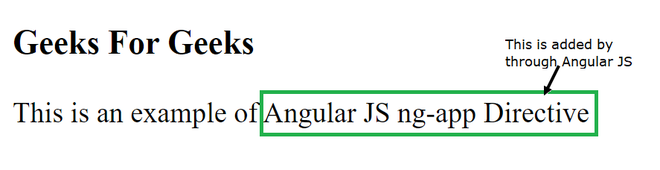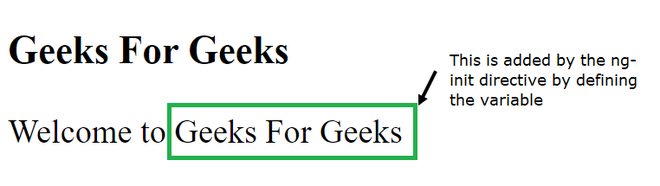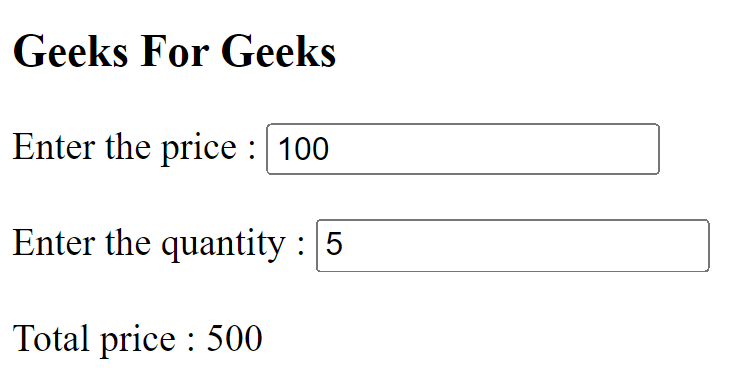Related Articles

# What is the role of ng-app, ng-init and ng-model directives in AngularJS ?

• Last Updated : 25 Jul, 2021

In this article, we will learn about the directives in AngularJS & explore the roles of various directives such as ng-app, ng-init & ng-model in angularJS.

The AngularJS directive is a command that gives HTML documents new functionality. When we use the AngularJS directives, it will first find all the directives in the HTML document and then parse the HTML document accordingly.

ng-app: The ng-app is the directive that is used to define the AngularJS application. By placing this in the HTML document, it indicates that this is an AngularJS application.

## HTML

 ```<``html``>`` ` `<``head``>``    ``<``title``>ng-app``    ``<``script` `src``=``"https://code.angularjs.org/1.6.9/angular.min.js"``>``    `````` ` `<``body``>``    ``<``h3``>Geeks For Geeks``    ``<``div` `ng-app``=``""``>``        ``This is an example of {{"Angular JS"``        ``+ "ng-app" + "Directive"}}``    `````` ` ``ng-init: The ng-init is the directive that is used for the initialization of the application data. In a simple way, it is used as a local variable in AngularJS. Sometimes we need some local data in your application, so by using the ng-init directive it can be achieved.

## HTML

 ```<``html``>`` ` `<``head``>``    ``<``title``>ng-init``    ``<``script` `src``=``"https://code.angularjs.org/1.6.9/angular.min.js"``>``    `````` ` `<``body``>``    ``<``h3``>Geeks For Geeks``    ``<``div` `ng-app``=``""` `ng-init``=``        ``"Website = 'Geeks For Geeks'"``>``        ``Welcome to {{Website}}``    `````` ` ``ng-model: The ng-model is the directive that is used to bind the value of the HTML control to application data. It is used to bind the data in the HTML document.

## HTML

 ```<``html``>`` ` `<``head``>``    ``<``title``>ng-model``    ``<``script` `src``=``"https://code.angularjs.org/1.6.9/angular.min.js"``>``    `````` ` `<``body``>``    ``<``h3``> Geeks For Geeks ``    ``<``div` `ng-app``=``""` `ng-init``=``"price=100; quantity=5"``>``        ``Enter the price : ``            ``<``input` `type``=``"text"` `ng-model``=``"price"``> ``            ``<``br``><``br``>`` ` `        ``Enter the quantity : ``            ``<``input` `type``=``"text"` `ng-model``=``"quantity"``> ``            ``<``br``><``br``>``        ``Total price : {{price * quantity}}``    `````` ` ``My Personal Notes arrow_drop_up